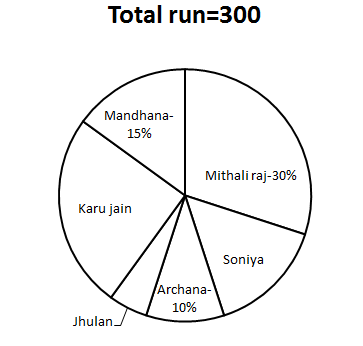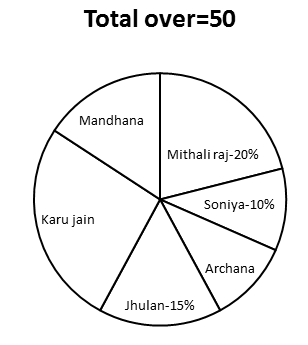# Crack IBPS PO : Data Interpretation : Aptitude day 60

Directions.1-5) The following Missing DI gives the details about six female cricket player’s percentage of total run and percentage of total over is given:

Pie chart 1 shows the percentage of total run scored by six female cricket players

Pie chart 2 shows the percentage of total over faced by six female cricket players

Strike rate=(Total runs scored)/(Total balls faced)×100Q.1) What is the strike rate of Karu jain if the central angle of total runs scored and total over of Karu jain is same which is 90 degree?

a) 600

b) 200

c) 100

d) Cannot be determined

e) None of these

Percentage of Karu Jain =100/360×90=25%

Total run scored by Karu Jain =25/100×300=75 runs

Total over for Karu Jain =25/100×50=12.5 over

Total balls faced by Karu Jain =12.5×6=75 balls

Strike rate of Karu Jain =75/75×100=100

Q.2) How many balls faced by Archana if the central angle of Archana’s total over is one by three times of Mithali raj central angle in total run?

a) 5

b) 30

c) 36

d) 6

e) None of these

Central angle of Mithali raj total run =360×30/100=108 degree

Central angle of Archana’s =1/3×108=36 degree

Percentage of Archana’s total over =100/360×36=10%

Total balls faced by Archana =10/100×50×6=30

Q.3) What is the difference between the strike rate of Mithali raj and Soniya if Soniya scores 50% of the run scored by Mithali raj?

a) 50

b) 0

c) 100

d) Cannot be determined

e) 150

Total run scored by Mithali raj =30/100×300=90 runs

Total over of Mithali raj =50×20/100=10 over (or)60 balls

Strike rate of Mithali raj =90/60×100=150

Total run scored by Soniya =50/100×90=45 runs

Total over of Soniya =50×10/100=5 over (or)30 balls

Strike rate of Soniya =45/30×100=150

Required difference =150-150=0

Q.4) What is the ratio between sum of the Jhulan’s and Archana’s runs to sum of the Mandhana’s and Karu jain’s runs, if Jhulan scores 50% of runs scored by Archana and Karu jain scores 30 runs more than of Mandhana’s total run?

a) 3:8

b) 2:5

c) 8: 3

d) 15:41

e) None of these

Archana’s total run =10/100×300=30 runs

Jhulan’s total run =30×50/100=15 runs

Mandhana’s total run =15/100×300=45 runs

Karu Jain’s total run =30+45=75 runs

Required ratio =(30+15):(45+75)=45:120=3:8

Q.5) What is the difference between the central angle of Mandhana total run and Archana’s total run? (In degree)

a) 36

b) 54

c) 90

d) 18

e) None of these

Mandhana’s central angle =360/100×15=54 degree

Archana’s central angle =360/100×10=36 degree

Required difference =54-36=18 degree

Directions.6-10) In the following question two quantities are given Quantity 1 and quantity 2. By solving those quantities then give corresponding answer.

Q.6)

Quantity 1: From a group of 5 male and 6 female, five persons are to be selected to form a committee so that at least 3 female are there in the committee. In how many ways can it be done?

Quantity 2: In how many different ways can the letters of the word ‘QUANTITATIVE’ be arranged in such a way that the vowels always comes together? (Repetition not allowed)

a) Quantity 1 ≥ Quantity 2

b) Quantity 1 = Quantity 2 (or) No relation

c) Quantity 1 > Quantity 2

d) Quantity 1 ≤ Quantity 2

e) Quantity 1 < Quantity 2

From quantity 1, Number of ways =5C2 ×6C3 +5C1×6C4+5C0×6C5/11C5 =281/462=281/462

From quantity 2, Number of ways =7!/(2!×3!×2!)=210

Quantity 1 < Quantity 2

Q.7)

Quantity 1: Three coins are tossed, what is the probability of getting at least two head?

Quantity 2: Two dice are thrown, what is the probability of getting sum of the two dice is multiple of 3?

a) Quantity 1 ≥ Quantity 2

b) Quantity 1 = Quantity 2 (or) No relation

c) Quantity 1 > Quantity 2

d) Quantity 1 ≤ Quantity 2

e) Quantity 1 < Quantity 2

From quantity 1, total possibility =2^3=8

Number of possibility =4

Required probability =4/8=1/2

From quantity 2, total possibility =6^2=36

Number of possibility =12

Total probability =12/36=1/3

Quantity 1 > Quantity 2

Q.8)

Quantity 1: The ratio between Siva’s present age and Mala’s present age is 2:3. After four years their ratio will become 7:10, what is the age of Mala 10 years ago?

Quantity 2: The average age of 20 girls in a class is two-third of the boys in a class and the average age of 36 boys in the class is four-fifth of the girls in the class, approximately what is the average age of the class?

a) Quantity 1 ≥ Quantity 2

b) Quantity 1 = Quantity 2 (or) No relation

c) Quantity 1 > Quantity 2

d) Quantity 1 ≤ Quantity 2

e) Quantity 1 < Quantity 2

From quantity 1,

(2x+4)/(3x+4)=7/10

20x+40=21x+28  =>x=12 years

Mala’s present age =3×12=36 years

Mala’s age 10 years ago =36-10=26 years

From quantity 2,

(20×2/3×36+36×4/5×20)/(20+36)=(480+576)/56~ 19 years

Quantity 1 > Quantity 2

Q.9)

Quantity 1: In a village 20000 people are living of that 20% migrated to another village and 50% of migrated people are unemployed, what is the total number of people who were employed in the migrated people?

Quantity 2: According to the report of Municipality government population 20% increases every year compared to the previous year, what is the total population in the year 2016, if the population in the year 2018 is 3600?

a) Quantity 1 ≥ Quantity 2

b) Quantity 1 = Quantity 2 (or) No relation

c) Quantity 1 > Quantity 2

d) Quantity 1 ≤ Quantity 2

e) Quantity 1 < Quantity 2

From quantity 1,

Number of employee people in the migrated =20000×20/100×50/100=2000

From quantity 2,

Number of people in 2018 =P(1+R/100)n

3600=P(1+20/100)2=>  3600×5/6×5/6=P

P = 2500

Quantity 1 < Quantity 2

Q.10)

Quantity 1: Vessel A contains milk and water in the ratio of 2:3 and vessel B contains Milk and Water in the ratio of 5:7, two vessels are mixed to make third vessel C, what percentage of water in the final mixture?

Quantity 2: There are two tanks P and Q having Alcohol and water in the ratio 1:4 and 3:5 respectively, two tanks mixed together and form a third tank S, what percentage of water in the final mixture?

a) Quantity 1 ≥ Quantity 2

b) Quantity 1 = Quantity 2 (or) No relation

c) Quantity 1 > Quantity 2

d) Quantity 1 ≤ Quantity 2

e) Quantity 1 < Quantity 2

From quantity 1,

Milk in vessel C =2/5+5/12=(24+25)/60=49/60

Water in vessel C =3/5+7/12=(36+35)/60=71/60

Milk and Water ratio in vessel C =49/60:71/60=49:71

Required percentage =71/120×100~ 59%

From quantity 2,

Alcohol in tank S =1/5+3/8=20/40

Water in tank S =4/5+5/8=(32+25)/40=57/40

Alcohol and Water in tank S =23/40:57/40=23:57

Required percentage =57/80×100~71%

Quantity 1 < Quantity 2